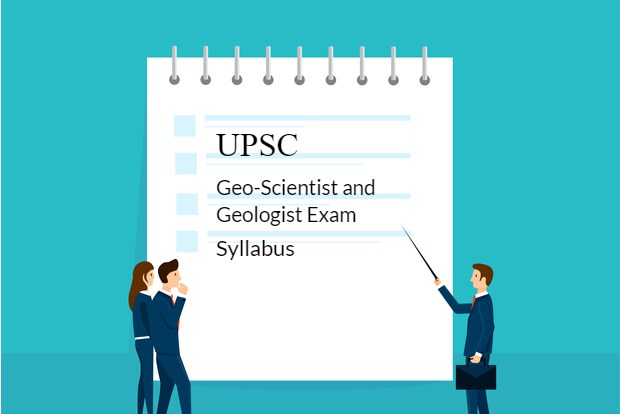# UPSC exam consists of three tiers: Best NDA Coaching In Lucknow## Exam pattern and syllabus for UPSC Geoscientist and Geologist exam:

Stage-I: Preliminary Examination

Stage-II: Main Examination

Stage-III: Personality Test## Jr Hydrogeologist:

• All the questions in the paper are conventional, that is each answer require essay style write up.
• The written exam comprises of 4 papers for every post and English being standard for each.
• Each subject paper contains 200 marks each.
• Exam duration is of 3 hours each.

## Syllabus:

### General English

1. Short essays
2. Conclusion
3. Tenses
4. Error correction
5. Articles
6. Vocabulary
7. Synonyms and Antonyms
8. Unseen passages
9. Comprehension
10. Sentence rearrangement
11. Verb
12. Fill in the blanks
13. Idioms and phrases
15. Theme detection
16. Word Formation
17. Subject-Verb Agreement
18. Grammar
19. Sentence completion
20. Passage completion

### Geology I

1. Geomorphology and remote sensing
3. Applied Geomorphology
4. Proterozoic stratigraphy
5. Zones: Brittle and Ductile shear zones
6. Gravity anomalies at mid-ocean ridges
7. Concepts of Magnetostratigraphy
8. Stratigraphy
9. Geodynamics
10. Palaeontology
11. Structural Geology

### Geology II

1. Mineralogy and Geochemistry & Isotope Geology.
2. Principles of optical mineralogy.
3. The external chemistry of crystals.
4. Introduction to mineralogy.
5. Accessory minerals.
6. Geochronology and age of the earth.
7. Igneous Petrology.
8. Origin of magmas.
9. Magmatic evolution and differentiation.
10. Magmatism and tectonics.
11. Intermediate rocks.
12. Petrogenetic provinces.
13. Metamorphic petrology & processes.
14. Concepts and Theory.
15. Effects of Metamorphism.
16. Metamorphism in space and time.
17. Environmental Geology and Natural hazards.
18. Air sampling techniques.
19. Fundamental concepts of Environmental Geology.

Geology III

1. Indian mineral deposits and mineral economics.
2. Ore genesis and Geophysics.
3. Mineral exploration.
4. Geology of fuels.
5. Engineering Geology.
6. Coastal geotechniques.
7. Mineralogy and geochemistry of radioactive minerals.
8. Maceral analysis of coal.
9. Characters of source and reservoir rocks.
10. Principles of mineral and prospecting and exploration.
11. Ore deposits and ore minerals.
12. Occurrence and distribution in India of metalliferous deposits.

### Geophysics I

1. Solid earth geophysics.
2. Earthquake and Engineering Seismology.
3. Mathematical methods in Geophysics.
4. Geophysical inversion.
5. Global optimization techniques.
6. Fundamental concepts of inverse physics.
7. Mathematical methods of physics.
8. Thermodynamics and Statistical physics.
9. Introductory atmospheric and space physics.

### Geophysics II

1. Geophysical potential fields (Gravity and Magnetic).
2. Electrical and Electromagnetic methods.
3. Seismic prospecting.
4. Borehole Geophysics (principals of well logging).
5. Atomic and molecular Physics and Properties and Characterization of materials.
6. Nuclear and Particle Physics.
7. Electromagnetic theory.
8. Classical Mechanics.
9. Electrostatics, Magneto statics.
10. Crystalline and amorphous structure of matter.
11. Airborne EM systems.
12. Principles of Gravity and Magnetic instruments.

### Geophysics III

1. Radiometric exploration / airborne geophysical surveys for Geological mapping.
2. Digital electronics, radar systems, Satellite communications.
3. Digital circuits. Number systems and codes.
4. Crystalline and Amorphous structure of matter.
5. Geophysical signal processing.
6. Remote sensing and GIS Applications.
7. Laser Cavity modes.
8. Semiconductor devices.
9. Laser systems.
10. Marine Geophysics.
11. Solid state physics.
12. Electronics and devices.
13. Quantum mechanics.

### Chemistry I

1. Chemical periodicity.
2. Acid-Base reactions.
3. Organometallic compounds.
4. Nuclear Chemistry.
5. p-Block Elements.
6. Chemical bonding and structure.
7. Chemistry and coordination compounds.
8. Precipitation and Redox reactions.
9. s-Block Elements.
10. Chemistry of d- and f- block elements.

### Chemistry II

1. Kinetic theory and gaseous states.
2. Collision of gas and molecules, real gases.
3. Thermodynamics and equilibrium.
4. Solution of non-electrolytes.
5. Adsorption and surface chemistry.
6. UV Spectra.
7. Application of Second Law of Thermodynamics.
8. Basic Principal and application of spectroscopy.
9. Chemical kinetics and catalysis.
10. Liquid state.
11. Acids- bases and solvents.
12. Thermodynamics
13. Quantum chemistry.
14. PMR Spectra.

### Chemistry III

1. Analytical chemistry.
2. Theoretical basis of quantitative inorganic analysis.
3. Gravimetric analysis.
4. Sampling and treating of samples for chemical analysis.
5. Volumetric analysis.
6. Acid base titration.
7. Redox Titration.
8. Complexometric titration.
9. Chromatographic methods of analysis.
10. X-ray methods of analysis.
11. Analysis of minerals. Ores and Alloys.
12. Basic organic chemistry.
13. Bonding and physical chemistry.
14. Mechanism of some name reactions.
15. Flame photometry and Atomic absorption spectrometry.
16. Inductively coupled plasma spectroscopy.
17. Analysis of petroleum and petroleum products.
18. UV-visible spectroscopy.
19. Electrocyclic reactions.
20. Organic reaction mechanisms.
21. Organometallic compounds.
22. Aldol and related reactions.
23. Organic spectroscopy.

### Hydrogeology

1. Origin, occurrence and distribution of water.
2. Groundwater Hydraulics.
3. Groundwater exploration and water well construction.
4. Geologic and hydrogeologic methods of exploration.
5. Groundwater quality.
6. Physical and chemical properties of water.
7. Groundwater management.